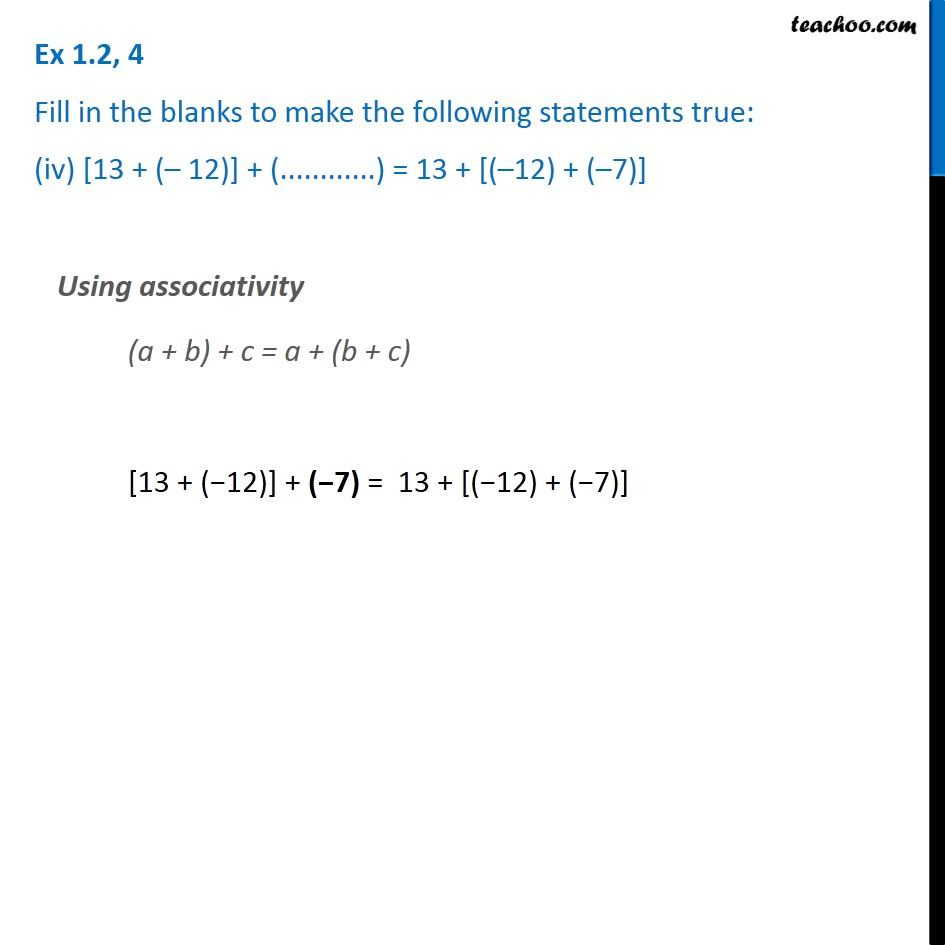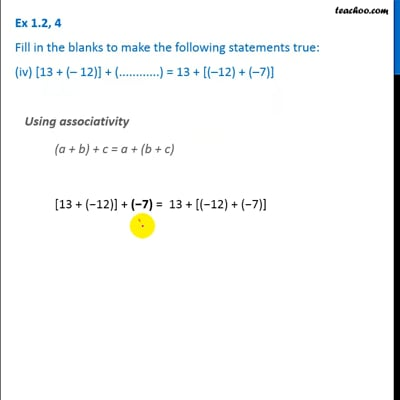Ex 1.2

Chapter 1 Class 7 Integers
Serial order wiseThis video is only available for Teachoo black users

Introducing your new favourite teacher - Teachoo Black, at only ₹83 per month

### Transcript

Ex 1.2, 4 Fill in the blanks to make the following statements true: (iv) [13 + (– 12)] + (............) = 13 + [(–12) + (–7)] Using associativity (a + b) + c = a + (b + c) [13 + (−12)] + (−7) = 13 + [(−12) + (−7)]